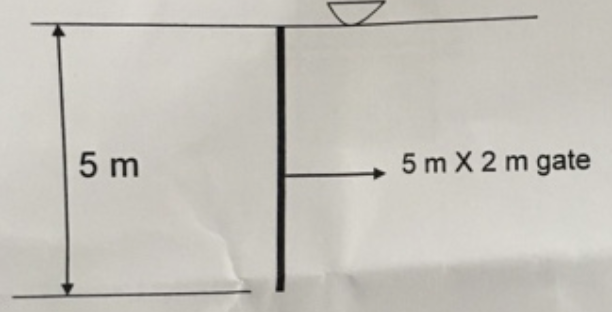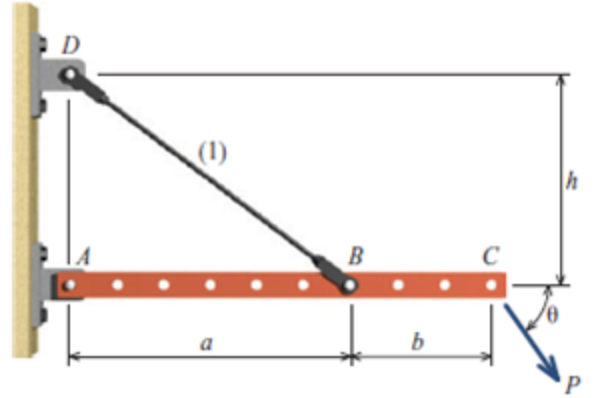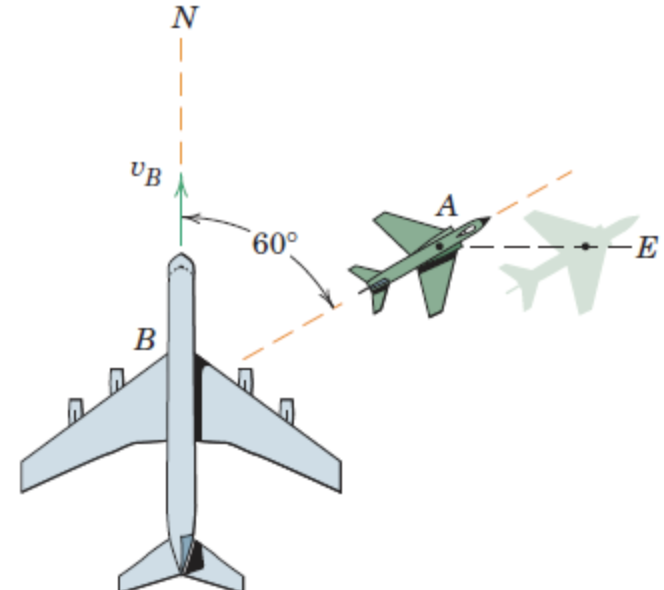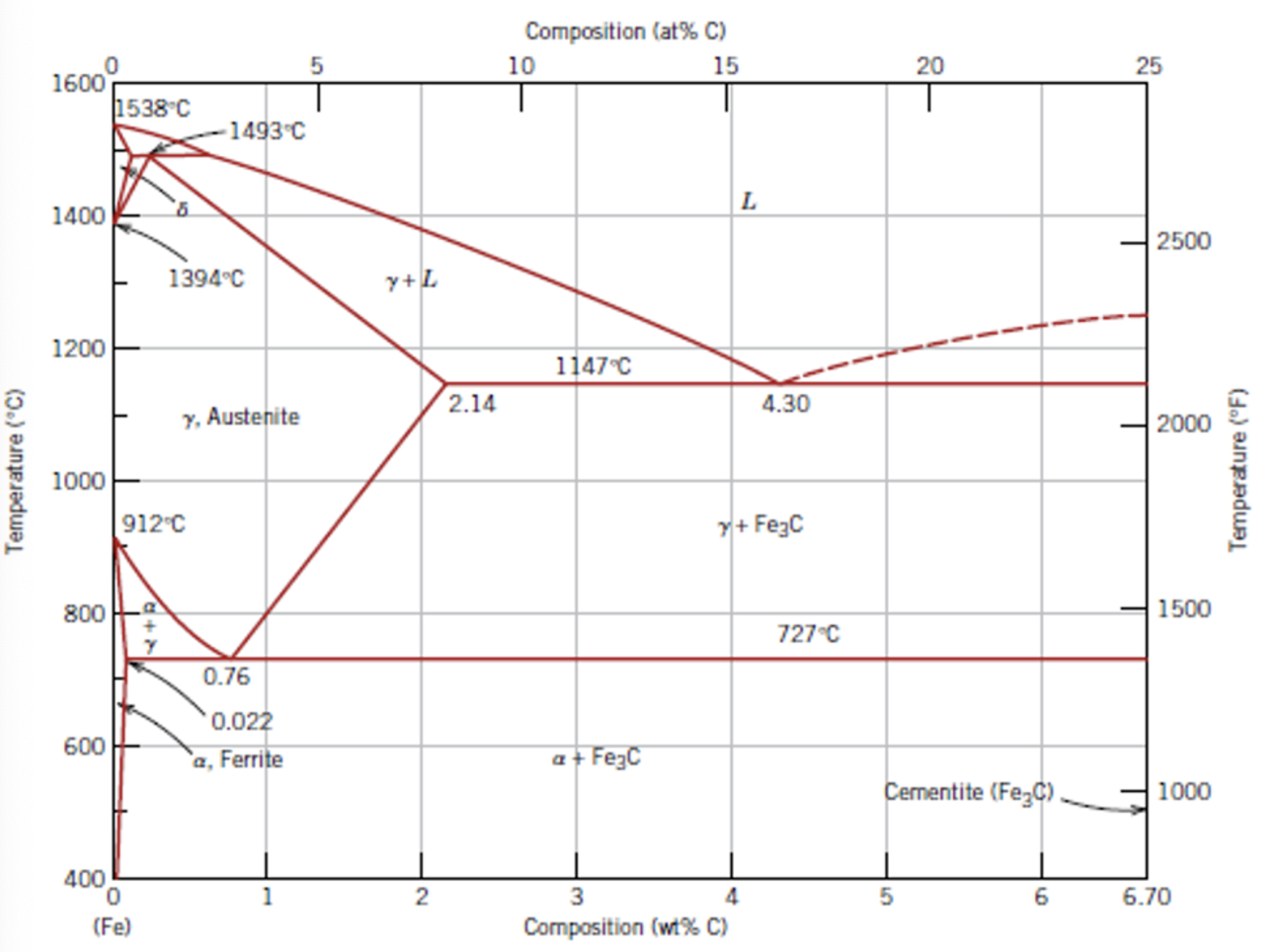##### Force acting

(a) How do you calculate hydrostatic force in gates?

(b) Calculate total hydrostatic force on the vertical gate. Also calculate the location of the hydrostatic force from topIn: Mechanical Engineering

##### Rigid bar ABC shown in Figure P1.30 is supported by a pin at bracket A and by tie rod (1).

Rigid bar ABC shown in Figure P1.30 is supported by a pin at bracket A and by tie rod (1). Tie rod (1) has a diameter of 5 mm, and it is supported by double-shear pin connections at B and D. The pin at bracket A is a single-shear connection. All pins are 7 mm in diameter. Assume a = 600 mm, b = 300 mm, h = 450 mm, P = 900 N, and θ = 55°. Determine the following:

(a) the normal stress in rod (1)

(b) the shear stress in pin B

(c) the shear stress in pin A

FIGURE P1.30In: Mechanical Engineering

##### The jet transport B is flying north with a velocity

The jet transport $$B$$ is flying north with a velocity $$v_{\mathrm{B}}=600 \mathrm{~km} / \mathrm{h}$$ when a smaller aircraft $$A$$ passes underneath the transport headed in the $$60^{\circ}$$ direction shown. To passengers in $$B$$, however, $$A$$ appears to be flying sideways and moving east. Determine the actual velocity of $$A$$ and the velocity which $$A$$ appears to have relative to $$B$$.In: Mechanical Engineering

##### Archimedes measured the weight of the crown in air to be 11.8 N and its weight in water to be 10.9 N. Was it pure gold?

It is said that Archimedes discovered the buoyancy laws when asked by King Hiero of Syracuse to determine whether his new crown was pure gold (SG = 19.3). Archimedes measured the weight of the crown in air to be 11.8 N and its weight in water to be 10.9 N. Was it pure gold?

In: Mechanical Engineering

##### Iron Carbon phase diagram

The phase diagram of Iron – Carbon:

Consider a 94 wt% Fe – 6 wt% C steel

at a temperature just below the eutectic point

shown in the Figure 9.24

for this problem.

(a)

Determine the compositions of cementite and austenite.

(b)

Determine the amount of cementite (in grams) that forms in 200g of steel.

(c)

Determine the amount of lamellar structure and pro-eutectic austenite in the 200g.In: Mechanical Engineering